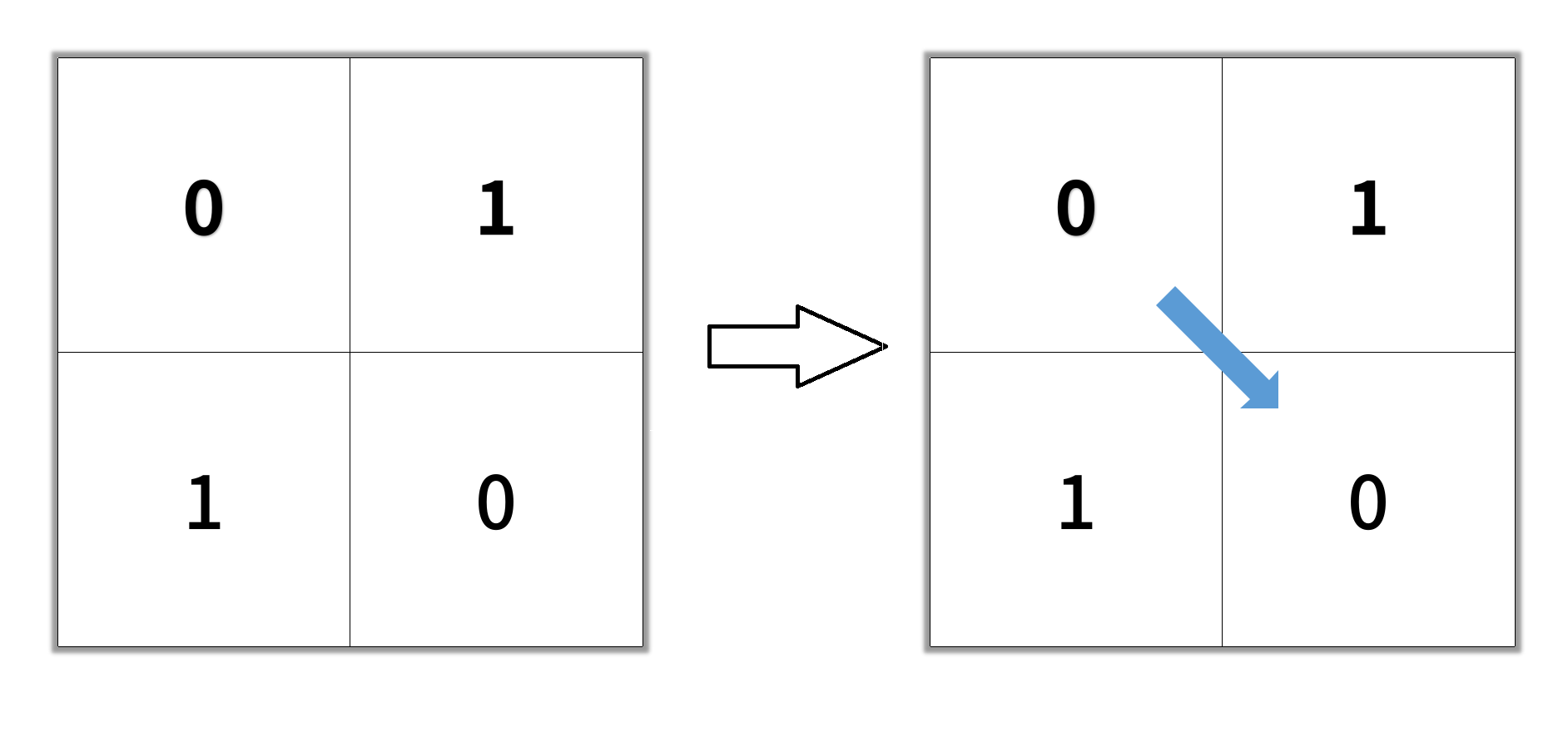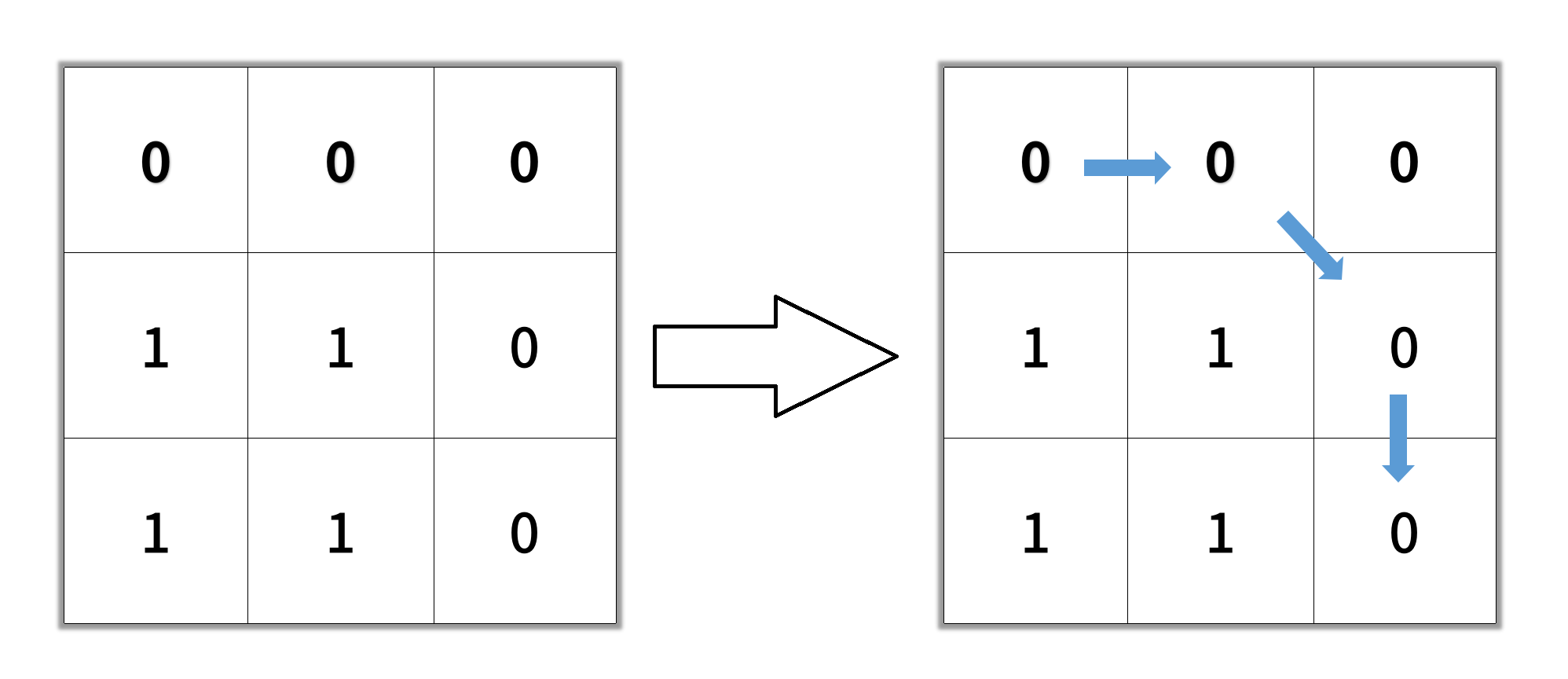## C# || How To Find The Shortest Clear Path In A Binary Matrix Using C#The following is a module with functions which demonstrates how to find the shortest clear path in a binary matrix using C#.

1. Shortest Path Binary Matrix – Problem Statement

Given an n x n binary matrix grid, return the length of the shortest clear path in the matrix. If there is no clear path, return -1.

A clear path in a binary matrix is a path from the top-left cell (i.e., (0, 0)) to the bottom-right cell (i.e., (n – 1, n – 1)) such that:

• All the visited cells of the path are 0.
• All the adjacent cells of the path are 8-directionally connected (i.e., they are different and they share an edge or a corner).

The length of a clear path is the number of visited cells of this path.

Example 1:``` Input: grid = [[0,1],[1,0]] Output: 2 ```

Example 2:``` Input: grid = [[0,0,0],[1,1,0],[1,1,0]] Output: 4 ```

Example 3:

``` Input: grid = [[1,0,0],[1,1,0],[1,1,0]] Output: -1 ```

2. Shortest Path Binary Matrix – Solution

The following is a solution which demonstrates how find the shortest clear path in a binary matrix.

``` 2. Shortest Path Binary Matrix - Solution C# // ============================================================================ // Author: Kenneth Perkins // Date: Jun 3, 2022 // Taken From: http://programmingnotes.org/ // File: Solution.cs // Description: Demonstrates how to find shortest path binary matrix // ============================================================================ public class Solution { // Search directions List<KeyValuePair<int, int>> directions = new List<KeyValuePair<int, int>>() { // Direction coordinates: (y, x) new KeyValuePair<int, int>(0, -1), new KeyValuePair<int, int>(0, 1), new KeyValuePair<int, int>(-1, 0), new KeyValuePair<int, int>(1, 0), new KeyValuePair<int, int>(1, 1), new KeyValuePair<int, int>(-1, -1), new KeyValuePair<int, int>(-1, 1), new KeyValuePair<int, int>(1, -1) }; public int ShortestPathBinaryMatrix(int[][] grid) { var NOT_VISITED = 0; var VISITED = 1; if (grid == VISITED) { return -1; } var queue = new Queue<KeyValuePair<int, int>>(); queue.Enqueue(new KeyValuePair<int, int>(0, 0)); grid = VISITED; var result = 0; while(queue.Count > 0) { ++result; for (var itemCount = queue.Count; itemCount > 0; --itemCount) { var current = queue.Dequeue(); // Get the current coordinates var row = current.Key; var col = current.Value; if (row == grid.Length - 1 && col == grid[row].Length - 1) { return result; } // Search in different directions foreach (var direction in directions) { var newRow = row + direction.Key; var newCol = col + direction.Value; if (IsValidCell(grid, newRow, newCol) && grid[newRow][newCol] == NOT_VISITED) { grid[newRow][newCol] = VISITED; queue.Enqueue(new KeyValuePair<int, int>(newRow, newCol)); } } } } return -1; } private bool IsValidCell(int[][] grid, int row, int col) { return row >= 0 && row < grid.Length && col >= 0 && col < grid[row].Length; } }// http://programmingnotes.org/ 123456789101112131415161718192021222324252627282930313233343536373839404142434445464748495051525354555657585960616263646566 // ============================================================================//    Author: Kenneth Perkins//    Date:   Jun 3, 2022//    Taken From: http://programmingnotes.org///    File:  Solution.cs//    Description: Demonstrates how to find shortest path binary matrix// ============================================================================public class Solution {    // Search directions    List<KeyValuePair<int, int>> directions = new List<KeyValuePair<int, int>>() {        // Direction coordinates: (y, x)        new KeyValuePair<int, int>(0, -1),        new KeyValuePair<int, int>(0, 1),        new KeyValuePair<int, int>(-1, 0),        new KeyValuePair<int, int>(1, 0),        new KeyValuePair<int, int>(1, 1),        new KeyValuePair<int, int>(-1, -1),        new KeyValuePair<int, int>(-1, 1),        new KeyValuePair<int, int>(1, -1)    };     public int ShortestPathBinaryMatrix(int[][] grid) {        var NOT_VISITED = 0;        var VISITED = 1;         if (grid == VISITED) {            return -1;        }         var queue = new Queue<KeyValuePair<int, int>>();        queue.Enqueue(new KeyValuePair<int, int>(0, 0));        grid = VISITED;         var result = 0;        while(queue.Count > 0) {            ++result;            for (var itemCount = queue.Count; itemCount > 0; --itemCount) {                var current = queue.Dequeue();                 // Get the current coordinates                var row = current.Key;                var col = current.Value;                 if (row == grid.Length - 1 && col == grid[row].Length - 1) {                    return result;                }                 // Search in different directions                foreach (var direction in directions) {                    var newRow = row + direction.Key;                    var newCol = col + direction.Value;                     if (IsValidCell(grid, newRow, newCol) && grid[newRow][newCol] == NOT_VISITED) {                        grid[newRow][newCol] = VISITED;                        queue.Enqueue(new KeyValuePair<int, int>(newRow, newCol));                    }                }            }        }        return -1;    }     private bool IsValidCell(int[][] grid, int row, int col) {        return row >= 0 && row < grid.Length && col >= 0 && col < grid[row].Length;    }}// http://programmingnotes.org/ ```

QUICK NOTES:
The highlighted lines are sections of interest to look out for.

The code is heavily commented, so no further insight is necessary. If you have any questions, feel free to leave a comment below.

Once compiled, you should get this as your output for the example cases:

``` 2 4 -1 ```

## C# || Palindromic Substrings – How To Find The Number Of Palindromic Substrings Using C#The following is a module with functions which demonstrates how to find the number of palindromic substrings using C#.

1. Count Substrings – Problem Statement

Given a string s, return the number of palindromic substrings in it.

A string is a palindrome when it reads the same backward as forward.

A substring is a contiguous sequence of characters within the string.

Example 1:

``` Input: s = "abc" Output: 3 Explanation: Three palindromic strings: "a", "b", "c". ```

Example 2:

``` Input: s = "aaa" Output: 6 Explanation: Six palindromic strings: "a", "a", "a", "aa", "aa", "aaa". ```

2. Count Substrings – Solution

The following is a solution which demonstrates how to find the number of palindromic substrings.

``` 2. Count Substrings - Solution C# // ============================================================================ // Author: Kenneth Perkins // Date: Jun 2, 2022 // Taken From: http://programmingnotes.org/ // File: Solution.cs // Description: Demonstrates how to find palindromic substrings // ============================================================================ public class Solution { public int CountSubstrings(string s) { var count = 0; for (var index = 0; index < s.Length; ++index) { count += CountPalindrome(s, index, index); // Count even sized count += CountPalindrome(s, index, index + 1); // Count odd sized } return count; } private int CountPalindrome(string s, int start, int end) { var count = 0; while (start >= 0 && end < s.Length && s[start--] == s[end++]) { ++count; } return count; } }// http://programmingnotes.org/ 12345678910111213141516171819202122232425 // ============================================================================//    Author: Kenneth Perkins//    Date:   Jun 2, 2022//    Taken From: http://programmingnotes.org///    File:  Solution.cs//    Description: Demonstrates how to find palindromic substrings// ============================================================================public class Solution {    public int CountSubstrings(string s) {        var count = 0;        for (var index = 0; index < s.Length; ++index) {            count += CountPalindrome(s, index, index);  // Count even sized            count += CountPalindrome(s, index, index + 1); // Count odd sized        }        return count;    }     private int CountPalindrome(string s, int start, int end) {        var count = 0;        while (start >= 0 && end < s.Length && s[start--] == s[end++]) {            ++count;        }        return count;    }}// http://programmingnotes.org/ ```

QUICK NOTES:
The highlighted lines are sections of interest to look out for.

The code is heavily commented, so no further insight is necessary. If you have any questions, feel free to leave a comment below.

Once compiled, you should get this as your output for the example cases:

``` 3 6 ```